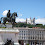## Thursday, July 26, 2007

### A counting gem

Consider the following puzzle:
Given 2n items, determine whether a majority element (one occuring n+1 times) exists. You are allowed one pass over the data (which means you can read the elements in sequence, and that's it), and exactly TWO units of working storage.
The solution is incredibly elegant, and dates back to the early 80s. I'll post the answer in the comments tomorrow, if it hasn't been posted already.

Update: A technical point: the problem is a promise problem, in that you are promised that such an element exists. Or, in the non-promise interpretation, you are not required to return anything reliable if the input does not contain a majority element.

1.Keep a count and store current value.

Pass over array, if you see the current stored value, inc count. Else decrement. If count reaches 0, replace the stored value with the current value in array.

In the end, you will end up with the right value.

2.This used to be a classic at informatics olympiads (I think I first saw it around 5th grade). Oh, the days... :)

-mip

3.A variation: now the sequence contains both arrival and departures of items: an arrival means that the count of the item increases by one, a departure means that it decreases by one. Assume (promise) that the net count of an item never dips below zero.

a) Again with the promise that a majority item exists, how much space is needed to find it?
b) Still using two units of working storage, determine whether there exists only a single item with non-zero count, and if so, return the item and its count.

Graham

4.Definition of "unit of workable storage" (whether it can store a value or number 0-n) would have been good... My solution uses one unit of each of those two types.

5."Given 2n items, determine whether a majority element (one occuring n+1 times) exists." OK, so it is a yes/no question.

Then there is the following piece of information: "you are promised that such an element exists."

OK, so I can solve this problem with zero units of storage area, and without inspecting the data at all: I just answer "YES" immediately.

6.MindHacks just recommended this interview on how to build a Cognitive Reserve and, who knows, probably solving all these puzzles help us...

http://www.sharpbrains.com/blog/2007/07/23/build-your-cognitive-reserve-yaakov-stern/

7.We can slide a window of 3 the n+1 times repeating element will appear twice in atleast one of the 2n-3+1 windows.

This will work if all the other elements are distinct.

8.This used to be a classic at informatics olympiads (I think I first saw it around 5th grade). Oh, the days... :)

Here is a different version of the problem:

9.BTW, a generalized version of this trick
was also used in "serious" research.

For instance,

http://www.cs.berkeley.edu/~christos/iceberg.ps

Yakov

10.I am curious about your elegant solution. Would you be kind enough to post it, if it is different from the first comment?

11.Okay, the following algorithm in Python code worked for me, it is similar to the first comment but not exactly.

An interesting variation on the algorithm is for a given proportion of maxima occurences (not 50+% but say 30+%), can you change the code with the same algorithm and make it work? [If it can be done then would it involve another computing a cnt comparision (instead of 0) to make it work]

# Pre tags not allowed in Blogger comments, so view source to copy the indented code.
import random

def maxima (rng):
"""Find the element with over 50% population occurence in range."""
max = rng
cnt = 0
for e in rng[1:]:
print max, e, cnt,
if e == max: cnt += 1
else: cnt -= 1

if cnt <= 0:
max = e
cnt = 0
print " -> ", max, e, cnt
if cnt > 0: return max

def populate (rng):
idx = range(len(rng))
while len(idx) > len (rng)/2 - 1:
x = random.randint(0,len(idx)-1)
rng[idx[x]] = random.randint(1,10)
del idx[x]
max = random.randint(1,10)
for x in idx:
rng[x] = max

rng = [None for i in xrange(100)]
populate (rng)
print maxima (rng), rng

12.So I should have clarified: the first comment is indeed the correct solution, and this can be generalized using k counters to get an element of frequency more than n/k.